Showing posts with label intermediate algebra. Show all posts
Showing posts with label intermediate algebra. Show all posts

## Saturday, April 20, 2019

### Free Algebra Study Guide with Videos

This free Algebra study guide is designed to supplement your current textbook. Your textbook is well written in a patient style and the appropriate sections should be read before each class meeting.
 Chapter 1 - Real Numbers and Their Operations    1.1 Real Numbers and The Number Line    1.2 Adding and Subtracting Integers    1.3 Multiplying and Dividing Integers    1.4 Fractions    1.5 Review of Decimals and Percents    1.6 Exponents and Square Roots    1.7 Order of Operations    1.8 Sample Exam Questions Chapter 2 - Linear Equations and Inequalities    2.1 Introduction to Algebra    2.2 Simplifying Algebraic Expressions    2.3 Linear Equations: Part I    2.4 Linear Equations: Part II    2.5 Applications of Linear Equations    2.6 Ratio and Proportion Applications    2.7 Introduction to Inequalities and Interval Notation    2.8 Linear Inequalities (one variable)    2.9 Review Exercises and Sample Exam Chapter 3 - Graphing Lines    3.1 Rectangular Coordinate System    3.2 Graph by Plotting Points    3.3 Graph using Intercepts    3.4 Graph using the y-intercept and Slope    3.5 Finding Linear Equations    3.6 Parallel and Perpendicular Lines    3.7 Introduction to Functions    3.8 Linear Inequalities (Two Variables)    3.9 Review Exercises and Sample Exam Chapter 4 - Solving Linear Systems    4.1 Solving Linear Systems by Graphing    4.2 Solving Linear Systems by Substitution    4.3 Solving Linear Systems by Elimination    4.4 Applications of Linear Systems    4.5 Solving Systems of Linear Inequalities (Two Variables)    4.6 Review Exercises and Sample Exam    [ Elementary Algebra Exam #1 Solutions ]    [ Elementary Algebra Exam #2 Solutions ]    [ Elementary Algebra Exam #3 Solutions ]    [ Elementary Algebra Exam #4 Solutions ]     --- EA Sample Final Exam Ch. 1-7 ---Chapter 5 - Polynomials and Their Operations    5.1 Rules of Exponents (Integer Exponents)    5.2 Introduction to Polynomials and Evaluating    5.3 Adding and Subtracting Polynomials    5.4 Multiplying Polynomials and Special Products    5.5 Dividing Polynomials  (Synthetic Division)    5.6 Negative Exponents and Scientific Notation    5.7 Review Exercises and Sample Exam Chapter 6 - Factoring and Solving by Factoring    6.1 Introduction to Factoring    6.2 Factoring Trinomials    6.3 Factoring Trinomials ax^2 + bx + c    6.4 Factoring Binomials    6.5 General Guidelines for Factoring Polynomials    6.6 Solving Equations by Factoring    6.7 Applications involving Quadratic Equations    6.8 Review Exercises and Sample Exam Chapter 7 - Rational Expressions and Equations    7.1 Simplifying Rational Expressions    7.2 Multiplying and Dividing Rational Expressions    7.3 Adding and Subtracting Rational Expressions    7.4 Complex Fractions    7.5 Solving Rational Equations    7.6 Applications of Rational Equations    7.7 Variation    7.8 Review Exercises and Sample Exam Do you find this cool math site helpful? If so please share the link or embed in your mylab math course. Also, feel free to copy-and-paste anything you find useful here.  All we ask is that you link back to this site:  OpenAlgebra.com

Intermediate Algebra (IA) (Algebra 2) topics below.

## Tuesday, July 23, 2013

### Solving Nonlinear Systems

In this guide, we have solved linear systems using three methods: by graphing, substitution, and elimination.  When solving nonlinear systems, we typically choose the substitution method, however, sometimes the other methods work just as well.  Remember that to solve a system of equations means to find the common points - if they exist. Given a system of equations consisting of a circle and a line then there can be three possibilities for solutions.  Remember that solutions to a system are ordered pairs (x, y). These will be the points where they intersect.
Solve the nonlinear systems.

Given a circle and a parabola there are five possibilities for solutions.
These nonlinear systems given algebraically might look like:
To find the points in common, we will usually use the substitution method. But, as in this case, the graphing and elimination method work just as well.

Solve.

Certainly there are many ways to solve these problems. Experiment with other methods and see if you obtain the same results.

Solve.

---

## Wednesday, May 8, 2013

### Intermediate Algebra (Algebra 2) Sample Exams

A complete set of sample exams covering topics found in the first 7 chapters of the online textbook Intermediate Algebra.

You will find mobile friendly solutions as well as links to printable copies in pdf format. Please feel free to copy and paste anything you find here into your LMS.

## Tuesday, May 7, 2013

### Hyperbolas

An hyperbola is the set of all points whose distances to two fixed points subtract to the same constant. In this section, we will focus on the equation of hyperbolas.The axis that contains the vertices is called the transverse axis and the axis that does not contain the vertices is called the conjugate axis. Also notice that the shape is very different than that of a parabola, hyperbolas are asymptotic to the following lines:We will make use of these asymptotes to sketch the graphs of hyperbolas.
[ Interactive: Hyperbolas ]
To easily graph the asymptotes of a hyperbola use the following process.

Step 1: Identify the center (h, k) from standard form.
Step 2: Plot points a units left and right from center.
Step 3: Plot points b units up and down from center.
Step 4: Draw the rectangle defined by these points.
Step 5: The lines though the corners of this rectangle are the asymptotes!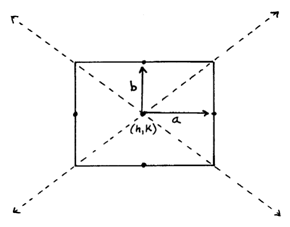Finally use the equation to determine if the parabola is vertical or horizontal.We knew to draw the above hyperbola opening to the left and right because the x^2 term was positive.  If the x^2 term is negative then the hyperbola opens up and down.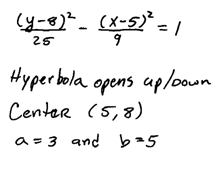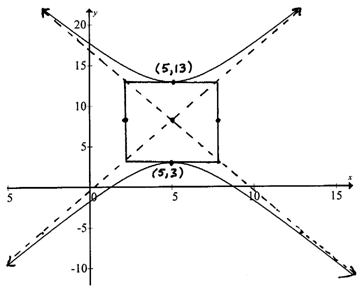Compare this hyperbola to the previous and note the difference in standard form. The rectangle and asymptotes are not actually part of the graph. We use these to obtain a more accurate sketch.  When graphed on a graphing utility the result looks like this.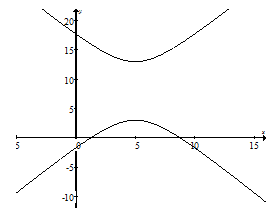Graph the hyperbola and give the equations of the asymptotes.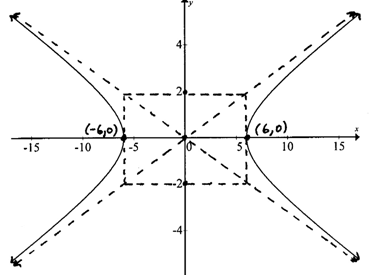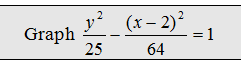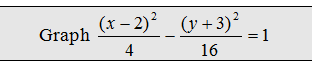The next examples require us to complete the square to obtain standard form.  Remember to factor the leading coefficient out of each variable grouping before using (B/2)^2 to complete the square.

Graph the hyperbola, x- and y- intercepts, and give the equations of the asymptotes.When we completed the square, notice that we added 64 and subtracted 225 to balance the equation.  It may appear, at first glance, that we added 16 and 25 to the left side. But in reality, after we distribute the 4 and -9 we added and subtracted larger values.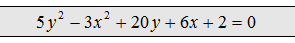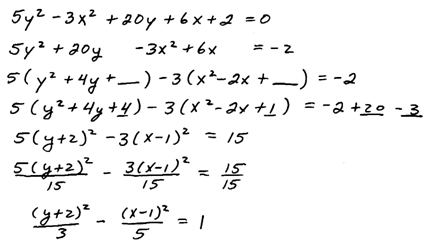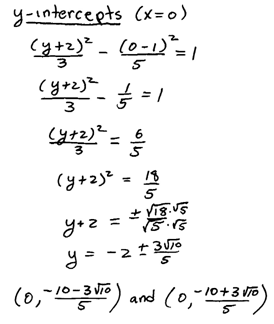When the transverse axis is vertical be careful with the center.  A common error is to use (-2, 1) for the center in the above example because the y variable comes first and we are used to reading these from left to right.  This would be incorrect so take care to use the correct h and k.

Example: Find the equation of the hyperbola centered at (-3, 1) where a = 2, b = 3, and with a vertical transverse axis.  Graph it.Example: Find the equation of the hyperbola with vertices (3,0) and (-3,0), horizontal transverse axis, and conjugate axis of length 4 units.Example: Find the equation of the hyperbola with center (2, -5), vertical transverse axis measuring 10 units, and conjugate axis measuring 6 units.YouTube Videos: Click problem to see it worked out.

---